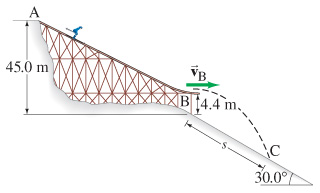# Problem: A 62-kg skier starts from rest at the top of a ski jump, point A in the figure , and travels down the ramp.If friction and air resistance can be neglected, determine her speed vB when she reaches the horizontal end of the ramp at B.Determine the distance s to where she strikes the ground at C.

###### FREE Expert Solution
89% (236 ratings)
###### Problem Details

A 62-kg skier starts from rest at the top of a ski jump, point A in the figure, and travels down the ramp.

If friction and air resistance can be neglected, determine her speed vB when she reaches the horizontal end of the ramp at B.

Determine the distance s to where she strikes the ground at C.

Frequently Asked Questions

What scientific concept do you need to know in order to solve this problem?

Our tutors have indicated that to solve this problem you will need to apply the Solving Projectile Motion Using Energy concept. You can view video lessons to learn Solving Projectile Motion Using Energy. Or if you need more Solving Projectile Motion Using Energy practice, you can also practice Solving Projectile Motion Using Energy practice problems.